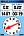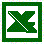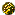# Route planningRoute planning calculation sheet for creating you own Marschskizze (Executable zip file, 100 kByte).
You can find the Instuctions and the Reduction method below the spreadsheet.

### Instructions:

#### Climbing speed

This is your climbing speed in meters/hour assuming good weather and snow conditions.
An average climbing speed is 300 meter/hour.
The climbing speed must be between 0 and 500 meter/hour.

#### Descending speed

This is your descending speed assuming good weather and snow conditions.
An average descending speed is 1000 meter/hour.
The descending speed must be between 0 and 5000 meter/hour.

#### Walking speed

Your walking speed assuming good weather and snow conditions.
An average walking speed is 4000 meter/hour.
The walking speed must be between 0 and 10.000 meter/hour.

#### Critical descending angle

The minimum steepness to be able to ski down instead of walking.
Under normal snow conditions 20 degrees is a good average.
The critical descending angle must be between 0 and 45 degrees.

#### Avalanche scale setting

The Avalanche scale setting must be 1, 2 or 3.
1 low, riskpotential=2
2 moderate, riskpotential=4
3 considerable, riskpotential=8.
For planning purpose it is best to select 3.
You will see an exclamation mark (in column Risk) in case the remaining risk is be below 1!

#### Waypoint *

Name of your waypoint. For example D3-A2-3 (Day 3, Alternative 2, 3th point).
The amount of characters can be limited by your GPS!

#### Description

For example "bridge over a stream".

GPS coordinates.

#### Altitude

Above see level in meters.

#### Ascending/Descending

Calculated fields (blue), difference between this and previous waypoint.

#### Distance *

Walking distance (horizontal) between this and previous waypoint.

#### Direction *

From previous- to this waypoint. Direction to walk in case you need to use your compass.

#### Estimated time

In case a proper time calculation cannot be made you can enter in this field your estimated time (in minutes).
For example when you expect technical difficulties. In all other cases you can leave it empty.

#### Time to next waypoint and accumulated time.

Time calculation based on the settings, meters to ascend or descend and the distance.

#### Orientation of the slope *

This is specially important in case the exposition of the hill is (partly) between WNW and E (292 <-> 90 degr.)
or even more important between NW en NO (315 <-> 45 degr.).
In all other case it does not influence the calculation of the "Reduction factor".

#### Angle of the slope *

This angle is used to calculate the risk. When it is not important, you can leave it empty.

#### Reduction factor

Not included is the reduction of the factors (see the Reduction method).Hills that are often used by skiers.50 meters of safety distance between each other.Small group. You can multiply the reduction factor with 2 if one of these counts. See for the calculation of the remaining risk paragraph 2.5.

#### Risk

As a warning, a exclamation mark will arise in case the remaining risk is >0 (based on avalanche scale setting)
Be aware that this reduction factor is calculated for just one slope orientation. Consider the worse case and be precise enough for reliable conclusions.

#### Point of No Return

Every point where you have to consider an option, like a crossing for an alternative route, is a Point of No Return.

#### Rows

Repaid the last altitude value on the remaining rows for a correct calculation of the total time. The other fields can be left empty for the remaining rows.

* This information can be easily obtained by using the OziExplorer mapping software

### Reduction method

 Avalanche scale Risk potential 1 (low) 2 2 (moderate) 4 3 (Considerable) 8

#### Reduction factors:

Every hills < 30º

6

Northface < 30º
Other hills < 40º

3

Every hill < 40º

2

1

or

Exposition of the hill not between NW and NE
2

Exposition of the hill not between WNW and E
3

2

3 Other (possible) reduction factors:Hills that are often used by skiers. (a lot of ski tracks after every snowfall)50 meters of safety distance between each other.Small group (3 or less)
2
2
2

#### Calculation of the risk:

##### Risk potential Remaining risk = -------------------------------------------------------------------- =  < 1                                Reduction factor x Reduction factor x (Reduction factor)

Calculate the remaining risk by dividing the Risk potential (see the avalanche bulletin) by the product of the reduction factors. As long we are going on slopes with a remaining risk smaller than 1 we will be on the save site.